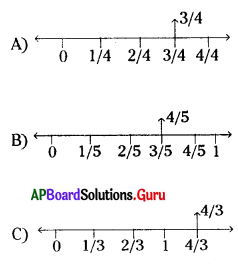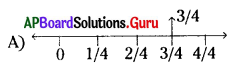Practice the AP 7th Class Maths Bits with Answers Chapter 2 Fractions, Decimals and Rational Numbers on a regular basis so that you can attempt exams with utmost confidence.

## AP State Syllabus 7th Class Maths Bits 2nd Lesson Fractions, Decimals and Rational Numbers with Answers

Question 1.
5 + $$\frac {8}{3}$$ =
A) $$\frac {13}{3}$$
B) 7$$\frac {2}{3}$$
C) $$\frac {7}{3}$$
D) 2$$\frac {9}{5}$$
B) 7 $$\frac {2}{3}$$

Question 2.
$$\frac {2}{3}$$ rd of a cake was cut and distributed. The rest of the part is
A) $$\frac{2}{3}$$
B) $$\frac{1}{3}$$
C) $$\frac{4}{3}$$
D) $$\frac{5}{3}$$
B) $$\frac{1}{3}$$Question 3.
8 × $$\frac{2}{5}$$ =
A) $$\frac{42}{5}$$
B) $$\frac{40}{5}$$
C) $$\frac{16}{5}$$
D) $$\frac{10}{5}$$
C) $$\frac{16}{5}$$

Question 4.
$$\frac{2}{3}$$ of $$\frac{5}{6}$$ =
A) $$\frac{7}{9}$$
B) $$\frac{7}{3}$$
C) $$\frac{7}{6}$$
D) $$\frac{10}{18}$$
D) $$\frac{10}{18}$$

Question 5.
Equalent fraction of $$\frac{4}{5}$$ is ……………..
A) $$\frac{16}{15}$$
B) $$\frac{12}{20}$$
C) $$\frac{20}{25}$$
D) $$\frac{28}{30}$$
C) $$\frac{20}{25}$$

Question 6.
$$\frac{11}{9}$$ = ……………… ?
A) $$\frac{121}{99}$$
B) $$\frac{55}{36}$$
C) $$\frac{44}{45}$$
D) $$\frac{77}{54}$$
A) $$\frac{121}{99}$$

Question 7.
3$$\frac{1}{3}$$ + 4 $$\frac{2}{3}$$ = ………………….?
A) $$\frac{1}{8}$$
B) 8
C) 7
D) $$\frac{22}{3}$$
B) 8

Question 8.
1 – 6 $$\frac{7}{8}$$ = …………………?
A) $$\frac{47}{8}$$
B) $$\frac{63}{8}$$
C) $$\frac{-45}{8}$$
D) $$\frac{-47}{8}$$
D) $$\frac{-47}{8}$$

Question 9.
The dimensions of one rectangle are 2$$\frac {1}{3}$$, 4$$\frac {1}{7}$$ then its area is ……………. sq. units.
A) $$\frac{28}{3}$$
B) $$\frac{29}{3}$$
C) $$\frac{31}{3}$$
D) $$\frac{29}{7}$$
B) $$\frac{29}{3}$$Question 10.
From the above sum the perimeter of a rectangle is …………….. units.
A) $$\frac{135}{27}$$
B) $$\frac{136}{27}$$
C) $$\frac{272}{21}$$
D) $$\frac{136}{21}$$
C) $$\frac{272}{21}$$

Question 11.
The total number of pages in a book are 7 $$\frac {1}{8}$$ . If Gayatri read 5$$\frac {1}{8}$$ pages, then number of pages are to be read?
A) 3
B) 2
C) 2$$\frac {1}{8}$$
D) 3$$\frac {1}{8}$$
B) 2

Question 12.
5$$\frac {7}{6}$$ × 1$$\frac {2}{37}$$ = ……………?
A) $$\frac{39}{37}$$
B) $$\frac{37}{39}$$
C) $$\frac{2}{13}$$
D) $$\frac{13}{2}$$
D) $$\frac{13}{2}$$

Question 13.
$$\frac {2}{5}$$ of 15 – $$\frac {1}{3}$$ of 12 = ……………?
A) 10
B) 0
C) 2
D) 1
C) 2

Question 14.
$$\frac {1}{4}$$ ×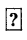= $$\frac {3}{20}$$
A) $$\frac{3}{5}$$
B) $$\frac{4}{5}$$
C) 3
D) $$\frac{1}{5}$$
A) $$\frac{3}{5}$$

Question 15.
Lowest form of $$\frac {2}{7}$$ × 3$$\frac {1}{2}$$ × 1$$\frac {1}{5}$$ × 2 $$\frac {1}{2}$$ = …………………?
A) 3
B) 1/2
C) 4
D) $$\frac{1}{3}$$
A) 3

Question 16.
2$$\frac{1}{3}$$ ÷ 3$$\frac{4}{7}$$ = ………………?
A) $$\frac{3}{25}$$
B) $$\frac{25}{3}$$
C) $$\frac{75}{49}$$
D) $$\frac{49}{75}$$
D) $$\frac{49}{75}$$Question 17.
The value of 23. 875 – 0.235 = ……………
A) 23.640
B) 21.640
C) 0.375
D) 25.105
A) 23.640

Question 18.
10.05 × 1.05 = ………………?
A) 10.5225
B) 10.525
C) 10.5525
D) 10.5252
C) 10.5525

Question 19.
A car uses 133 litre’s of petrol to run 190 kms. Then number of Its of petrol to run
110 kms is ………………..?
A) 77 litre’s
B) 87 litre’s
C) 66 litre’s
D) 63 litre’s
A) 77 litre’s

Question 20.
Which is greater
$$\frac{7}{4}, \frac{9}{4}, \frac{8}{4}, \frac{11}{4}$$
A) $$\frac{7}{4}$$
B) $$\frac{9}{4}$$
C) $$\frac{8}{4}$$
D) $$\frac{11}{4}$$
D) $$\frac{11}{4}$$

Question 21.
Which is lesser $$\frac{3}{5}, \frac{2}{5}, \frac{7}{5}, \frac{6}{5}$$?
A) $$\frac{3}{5}$$
B) $$\frac{2}{5}$$
C) $$\frac{3}{5}$$
D) $$\frac{3}{5}$$
B) $$\frac{2}{5}$$

Question 22.
Arrange in ascending order $$\frac{2}{3}, \frac{5}{3}, \frac{6}{5}$$
A) $$\frac{2}{3}, \frac{5}{3}, \frac{6}{5}$$
B) $$\frac{5}{3}, \frac{2}{3}, \frac{6}{5}$$
C) $$\frac{2}{3}, \frac{6}{5}, \frac{5}{3}$$
D) $$\frac{6}{5}, \frac{5}{3}, \frac{2}{3}$$
C) $$\frac{2}{3}, \frac{6}{5}, \frac{5}{3}$$

Question 23.
Which of the following fraction is greatest?
A) $$\frac{1}{2}$$
B) $$\frac{2}{5}$$
C) $$\frac{5}{8}$$
D) $$\frac{1}{3}$$
C) $$\frac{5}{8}$$Question 24.
Simplest form of $$\frac{\mathbf{3 7 5}}{\mathbf{1 0 0 0}}$$ is ………………
A) $$\frac{3}{8}$$
B) $$\frac{8}{3}$$
C) $$\frac{5}{8}$$
D) $$\frac{3}{5}$$
A) $$\frac{3}{8}$$

Question 25.
Which of the following is not correct (not equal)?
A) $$\frac{2}{3}=\frac{84}{75}$$
B) $$\frac{3}{8}=\frac{375}{1000}$$
C) $$\frac{70}{10}=\frac{7000}{1000}$$
D) $$\frac{25}{45}=\frac{125}{175}$$
A) $$\frac{2}{3}=\frac{84}{75}$$

Question 26.
$$\frac {4}{7}$$ ×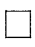= $$\frac {32}{35}$$
A) $$\frac{8}{7}$$
B) $$\frac{8}{5}$$
C) $$\frac{7}{5}$$
D) $$\frac{7}{8}$$
B) $$\frac{8}{5}$$

Question 27.
$$\frac{-12}{51}=\frac{(\mathbf{x})}{68}$$ ⇒ x = ………………..?
A) 16
B) 48
C) – 48
D) – 16
D) – 16

Question 28.
Which of the following statement is false?
A) Every rational number is an integer.
B) A rational number is in the form of p/q, q≠0
C) $$\frac{5}{7}, \frac{6}{11}, \frac{15}{11}$$ are equalent fractions.
D) $$\frac{44}{48}=\frac{77}{96}$$
D) $$\frac{44}{48}=\frac{77}{96}$$

Question 29.
$$\frac {11}{9}$$ is a ……………… fraction.
A) proper
B) an improper
C) mixed
D) none
B) an improper

Question 30.
The reciprocal of an improper fraction is a …………………. fraction.
A) proper
B) an improper
C) mixed
D) none
A) properQuestion 31.
In 8376.705 the digit is in Hundreth place is ………………..
A) 7
B) 5
C) 0
D) 3
C) 0

Question 32.
The place value of “tenths” digit in, 763.128 is ……………..
A) 8
B) 1
C) 2
D) 6
B) 1

Question 33.
The place value of the digit ‘7’ in 19.171 is ………………?
A) tenths
B) hundreths
C) thousandths
D) tens
B) hundreths

Question 34.
A number which in the form of p/q is called a ……………. number.
A) irrational
B) rational
C) real
D) integer
B) rational

Question 35.
$$\frac{123}{1000}$$ =
A) 1.23
B) 12.3
C) 0.123
D) 1230
C) 0.123

Question 36.
1827.582 × 10 =
A) 18.7582
B) 182758.2
C) 18275.82
D) None
C) 18275.82

Question 37.
3.82 × 100 =
A) 382
B) 38.2
C) 0.0382
D) 3820
A) 382Question 38.
Decimal form of 99 rupees 77 paise is ………………
A) 9.977
B) 99.77
C) 0.9977
D) 997.7
B) 99.77

Question 39.
2407 gms = ……………… kgs.
A) 2.407
B) 24.07
C) 0.2407
D) 240.7
A) 2.407

Question 40.
Expanded form of 1.11 is …………………
A) 1 × 1 + $$\frac{1}{100}$$ + $$\frac{1}{1000}$$
B) 1 × 10 + $$\frac{1}{10}$$ + $$\frac{1}{100}$$
C) 1 × 1 + $$\frac{1}{10}$$ + $$\frac{1}{1000}$$
D) 1 × 1 + $$\frac{1}{10}$$ + $$\frac{1}{100}$$
D) 1 × 1 + $$\frac{1}{10}$$ + $$\frac{1}{100}$$

Question 41.
43.071 × 100 = ……………….?
A) 43071
B) 0.43071
C) 4307.1
D) 430.71
C) 4307.1

Question 42.
Standard form of 9 × 10 + 8 × $$\frac{1}{10}$$ + 7 × $$\frac{1}{100}$$ + 6 × $$\frac{1}{1000}$$ = ………………?
A) 98.76
B) 9.0876
C) 9.876
D) 98.076
B) 9.0876

Question 43.
95 mm = ……………… kms.
A) 0.000095
B) 0.00095
C) 0.0095
D) 0.95
A) 0.000095

Question 44.
Which of the following shaded region represents 3/4th fraction.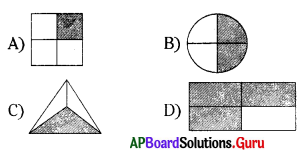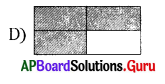Question 45.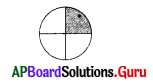The shaded region represents ……………..
A) $$\frac{1}{2}$$
B) $$\frac{1}{3}$$
C) $$\frac{1}{4}$$
D) $$\frac{1}{5}$$
C) $$\frac{1}{4}$$

Question 46.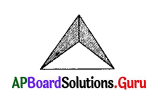The shaded region represents ……………..
A) $$\frac{2}{3}$$
B) $$\frac{3}{2}$$
C) $$\frac{1}{2}$$
D) $$\frac{1}{3}$$
A) $$\frac{2}{3}$$

Question 47.
PictorIal representation of 3 × $$\frac{1}{4}$$ = $$\frac{3}{4}$$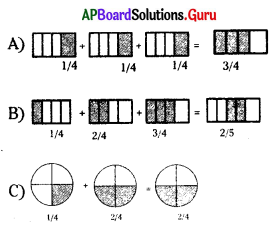D) None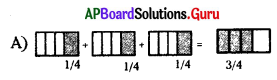Question 48.
Which of the following are not equal?
A) $$\frac{-2}{3}, \frac{-4}{6}$$
B) $$\frac{-1}{2}, \frac{-8}{6}$$
C) $$\frac{-1}{2}, \frac{-8}{16}$$
D) $$\frac{-1}{4}, \frac{-2}{8}$$
B) $$\frac{-1}{2}, \frac{-8}{6}$$